Dept. of Physics
Shri Chhatrapati Shivaji College, Omerga-413606, India
UniPhysics

I think that the emergence and development of modern physics was a giant leap in the history of mankind. This is because the main theories of modern physics reshaped our perception of the universe and caused an incredible scientific revolution. It is based on the two major breakthroughs of the twentieth century: relativity and quantum theory. Aim of the UniPhysics website is to reconsider the origin of quantum theory for discussion. It is devoted to solve the unsolved problems by classical physics. It believes on one physics and does not believes on bifurcation of physics such as classical and quantum. In universe, one thing cannot have two tempers simultaneously.

#### Classical explanation of photoelectric effect:

I think Einstein’s explanation of photoelectric effect were particularly responsible to develop the theory of quantum mechanics. While explaining the photoelectric effect he proposed that light consists of tiny packets of energy known as photons or light quanta. Each packet carries energy hv that is proportional to the frequency v of the corresponding electromagnetic wave. Thus he proposed the duel nature of light - wave as well as particle.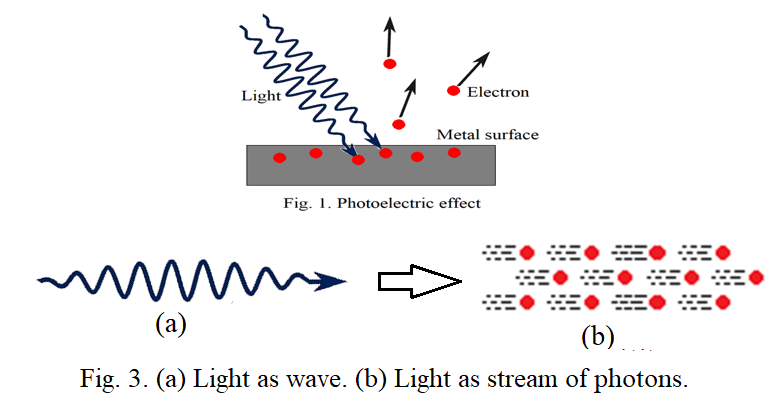Obviously, once if we accept wave can behave as a particle then a particle should also behave as a wave. That point was raised by deBroglie and proposed the duel nature of matter which reshaped our perception about the universe. Actually wave nature and particle nature are very different from each other. Why people have accepted the duel nature of light is beyond my imagination. I suppose explanation of photoelectric effectusing light as electromagnetic wave is possible. In classical physics one thing which we have left is the asymmetric electric field and asymmetric electric force . The asymmetric electric force applied by the electric field in the light wave is responsible to produce the photoelectric effect. It is explained on ‘Photoelectric effect’page of this website. The asymmetric electric force is responsible to produce an apparent force which is proportional to the frequency of the light wave because of which the kinetic energy of the photoelectrons is found to be proportional to the frequency. The properties of the apparent force are similar to the magnetic force suggesting that the magnetic force produced due to an electromagnetic wave increases with increase in frequency of the wave. It can be experimentally proved . This dependence cannot be supported by the formal classical electrodynamics. Further this discussion suggests that there should be a phase difference of 90 degree between electric field wave and magnetic field wave which is also not supported by the usual classical electrodynamics.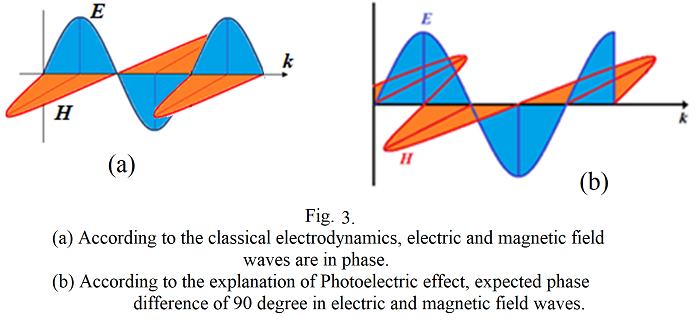#### Total length of single light wave emitted by an atom:

In lower physics divisions, generally we do Newton’s ring experiment to calculate the wavelength of sodium light. But we ignore on the side that how many rings are to be formed. What is the meaning of the formation of number of rings? Actually if ‘n’ number of rings are formed indicates that ‘n’ number of wavelengths contains in a single light wave emitted by sodium atom. In newton’s ring experiment, suppose we count 200 rings indicates that the total length of single light wave emitted by sodium atom is at least 200 times the wavelength which comes approximately 117860nm. This is very long wave. How it can act as a single photon and how it can be absorbed by a single electron is beyond our imagination. Further, transition of electron, in sodium atom, from higher energy level to lower energy level produces a wave containing 200 wavelengths then the corresponding electron should be oscillated 200 times with the frequency of the wave while producing it. It means we don’t know yet the complete emission mechanism of EM wave of atoms. We have used air-wedge method, as it is better than to Newton’s ring experiment, to count the fringes which is discussed in ‘total length of single light wave emitted by atom’ page.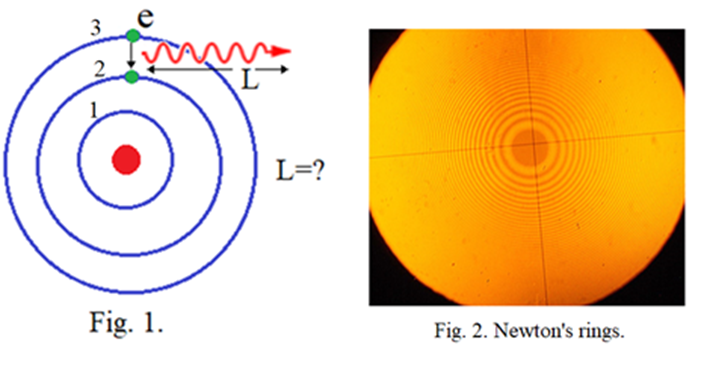#### What is magnetic field?

Explanation of photoelectric effect says that the electric field in EM wave is asymmetric at major times and produces asymmetric electric force which is responsible to produce an apparent force. The apparent force is proportional to the frequency of the EM wave and can be the true nature of magnetic force. Therefore, the magnetic force produced by an EM wave should increase with increase in frequency of the wave which can be verified experimentally as suggested in ‘What is magnetic field?’ page. It gives answer to why magnetic monopoles are absent in the universe.it will change completely the base of electrodynamics.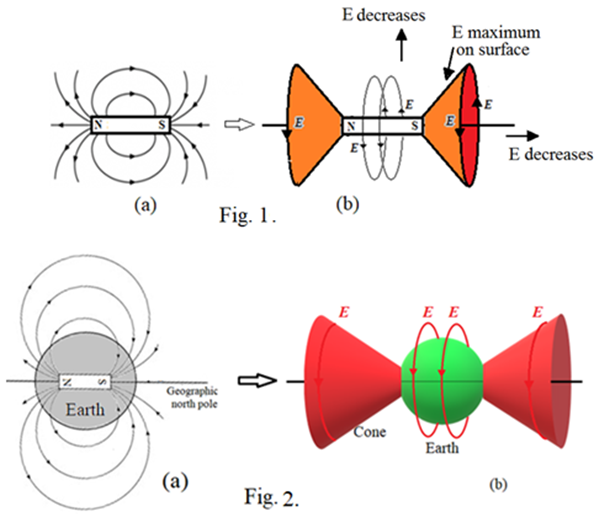#### Spin atomic model:

We have seen by Newton’s ring experiment or air-wedge method experiment that, while transition ofan electron from higher energy level to lower energy level in sodium atom, produces an electromagnetic wave containing more than 500 wavelengths indicates that the corresponding electron should be oscillated more than 500 times while producing the wave. It implies that the electrons in the atom may not be revolving around its nucleus. How electrons can maintain their safe distance from the nucleus in atoms using their spin motions without revolving in orbits or without acting as wavesis explained in ‘Atomic structure’ page. This atomic model supports to the formation of molecules, compounds and solid materials by atoms.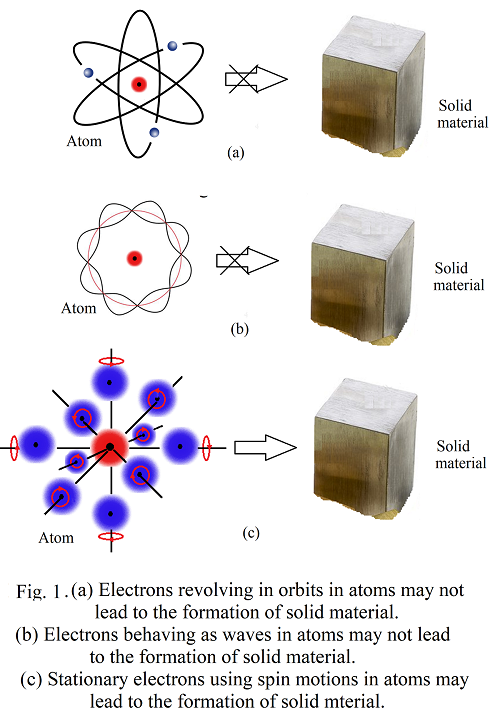Reader’scomments and feedback are welcomed and appreciated. I wanted to thank those of you who have visited to this website. I hope that you are finding some helpful information that may be changing our perception of the universe. It will bring us to the ground. I request all of you to be a part of building the ‘UniPhysics’. Your valuable comments and suggestions will give right direction to this movement. I request readers to email their comments and suggestions on ghjadhav@uniphysics.org.in that will be placed on ‘Reader’s Comments’ page.

## Classical explanation of photoelectric effect: Magnetic force should increase with increase in frequency of EM waves

#### Photoelectric effect:

The phenomenon of ejection of electrons from a metal surface when light of suitable frequency strikes on it.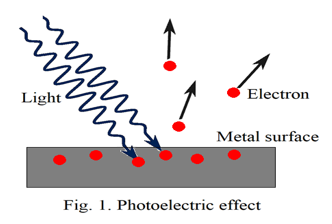#### Important Characteristic of the photoelectric effect:

The kinetic energy of the emitted electron is proportional to the frequency of the incident light.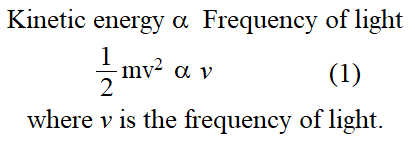#### Failure of classical mechanics:

The photoelectric effect is generally considered to be a discovered by Hertz’s (1887). According to the contemporary knowledge, light is an Electromagnetic wave.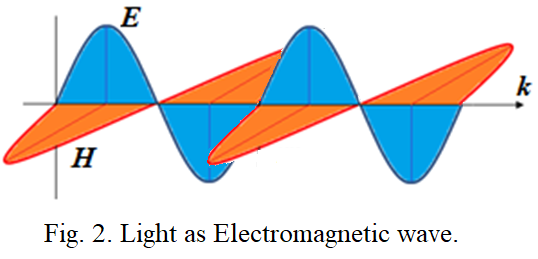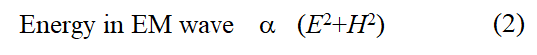It means energy is proportional to the intensity of light. It does not involve the frequency of light. Therefore, the classical electrodynamics is not able to explain the photoelectric effect. Until 1905, that crisis was not solved successfully by anyone.

#### Einstein’s explanation on photoelectric effect:

To the surprise, Einstein had published his paper in 1905 providing revolutionary explanation on the photoelectric effect. It was based on his “light quanta” hypothesis. He proposed that the light behaves as a stream of independent, localized units of energy (light quanta further called as photons). Each photon has an energy hv where v is the frequency of the light wave and h is the plank’s constant. A photon could be absorbed by a single electron imparting to it all its energy in photoelectric effect yielding the following equation,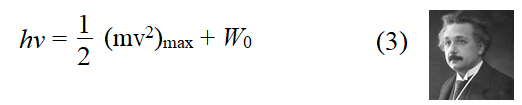where W0 is the work function of the metal.

Obviously eq. (3) obeys all the laws of photoelectric effect. It seemed that the crisis of photoelectric effect was solved. But few things are yet to be answered.

We don’t know.

#### An electron absorbs energy of a photon means what, do we have clear idea?

We don’t have really.

#### If a mass particle is at rest, what are the essentials to displace it from its position?

Generally, either a force is required or a transfer of momentum.

#### What is happening at the position of the electron while getting ejected in the photoelectric effect?

We don’t know exactly.

#### Can photoelectric effect happen if there is a charge-less particle at position of the electron?

We don’t know exactly but should not happen. Charge of the electron must be playing important role in this effect but we don’t know exactly. Einstein ignored charge on the electron. We don’t know about all such things which are essential and important to understand the photoelectric effect. Unfortunately no one is pointing on these. We are proceeding further such as all things are well explained by Einstein. We believe that Einstein could understand the photoelectric effect, specially the duel nature of light, and is beyond imagination of common man. But we should not go like that. Science is not a way to believe. It is to test and understand in all ways. It is to be answered and satisfied in all ways. Einstein’s hypothesis of “light quanta” was not taken seriously by mathematically adept physicists for just over fifteen years. The reasons are clear. It seemed to be an unnecessary rejection of the highly verified classical theory of radiation. How “light quanta” could possibly explain interference phenomena was always the central objection. There is a big difference between the two natures.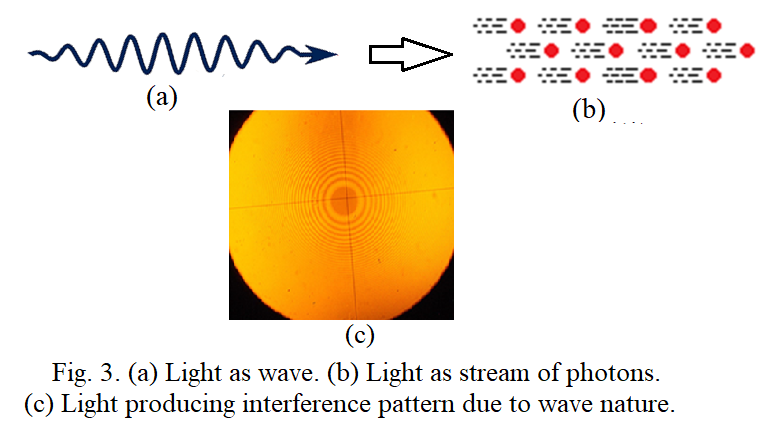Though Einstein proposed the concept of ‘light quanta’ but he couldn’t understand it in his whole life. In 1954 he wrote to his friend Michael Besso expressing his frustration;

“All these fifty years of conscious brooding have brought me no nearer to the answer to the question, ‘What are light quanta?’ Nowadays every Tom, Dick and Harry thinks he knows it, he is mistaken.”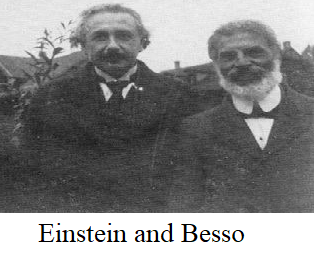Einstein couldn’t understand which force is responsible to eject electrons in the photoelectric effect. Let us investigate step by step which force can be responsible to produce the photoelectric effect. For investigation, we consider light is an electromagnetic wave of particular frequency. Further, as electron bears electric charge, therefore, electromagnetic force applied by the light wave on the electrons must be responsible to produce the effect.

#### Reinvestigating the Photoelectric Effect:

Light consist of electromagnetic waves propagating with speed c in vacuum with having electric and magnetic field structure as illustrated in Fig. 4(a). To understand the photoelectric effect with this structure, we examine hypothetically such types of one wave incident on a metal surface.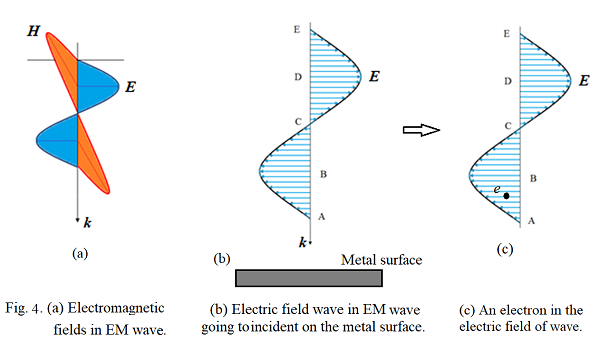At first we examine the effect of electric field in the wave on the electrons at the metal surface (Fig. 4(b)). As time advances, it progressively interacts with the metal surface and hence with electrons at it. We divide the field into four regions AB, BC, CD and DE. The electric field value at position A is zero producing no force on the subjected electrons. As the wave advances, the field strength subjected to the electron increases. We wish to examine the instant when the subjected electron is at the center of the region AB as illustrated by Fig. 4(c). This electric field exerts an electric force on the electron proportional to the electric field strength at its position in opposite direction of the field. One thing, here, to consider is that the electric field of the wave is not symmetric about the line of force exerted on the electron. Under this situation, the electron cannot be pushed only along the direction of the force eventually the force equation, F = qE, may not work properly. To understand the force action more elaborately, we have to take into consideration the electric field of the electron in addition to the applied electric field of the wave and visualize the existence of the electric force in terms of field-field interaction which can be explained as follows.

#### Force through field-field interaction:

Case (1): In fig. 5(a), the force between the two charges is expressed in terms of their charges. It may be considered as force in terms of charge-charge interaction.

Case (2): In fig 5(b), charge q1 produces electric field E1 around itself. If another charge q2 is brought into the field then the field applies force on the charge. It may be considered as force in terms of field-charge interaction. The force equation is given by F2 = q2E1, where E1 is the electric field produced by charge q1 at position of charge q2. Force is mutual. Therefore, charge q2 also applies force on charge q1 in terms of field-charge interaction by F1 = q1E2, where E2 is the electric field produced by the charge q2 at position of charge q1.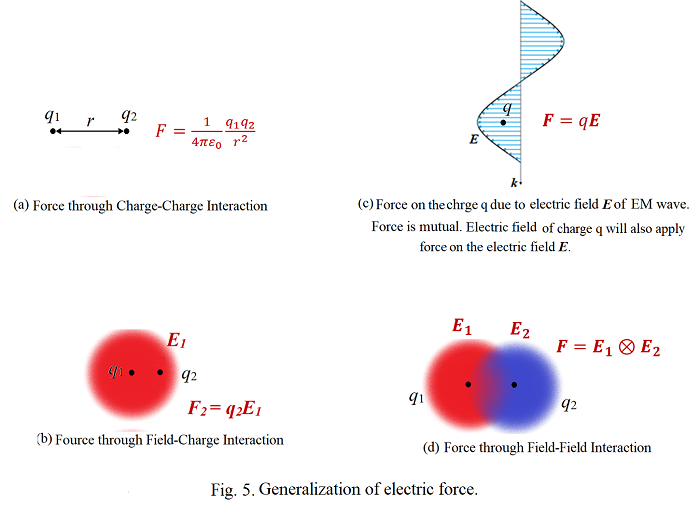Case (3): In fig. 5(c), an electric field wave in an electromagnetic wave is propagating with speed of light and interacts with a charge q at an instant. This field also applies force on the charge in terms of field-charge interaction. If E is the electric field of the electric wave at position of the charge q at any instant then the force equation is F = qE. But the force is mutual. Hence the charge q should also apply force on the electric field of the wave. As there is no charge associated with electric field of the wave, the electric field of the charge q must apply force on the electric field of the wave which may be considered as force through field-field interactions. Obviously, as the subjected charge q is always associated with its own electric field, therefore, the electric field of the applied wave must apply force on the field of the charge resulting into the force through field-field interactions. Thus finally one can conclude that the electric force exists not in terms of charge-charge interaction or field-charge interaction but it exists in terms of field-field interactions.

Case (4): In fig. 5(d), if two charges are brought close to each other, then force between them, either attractive or repulsive, is existed in terms of their field-field interactions. It means the electric field of first charge exerts force on the electric field of the second charge and vice versa. Thus a new electric force equation is to be developed which could explain the force in terms of the field-field interaction. At present we consider existence of electric force in terms of the field-field interactions for further discussion.Fig 4(c) is more elaborated in fig. 6 where the effect of electric force through field-field interactions can be understood.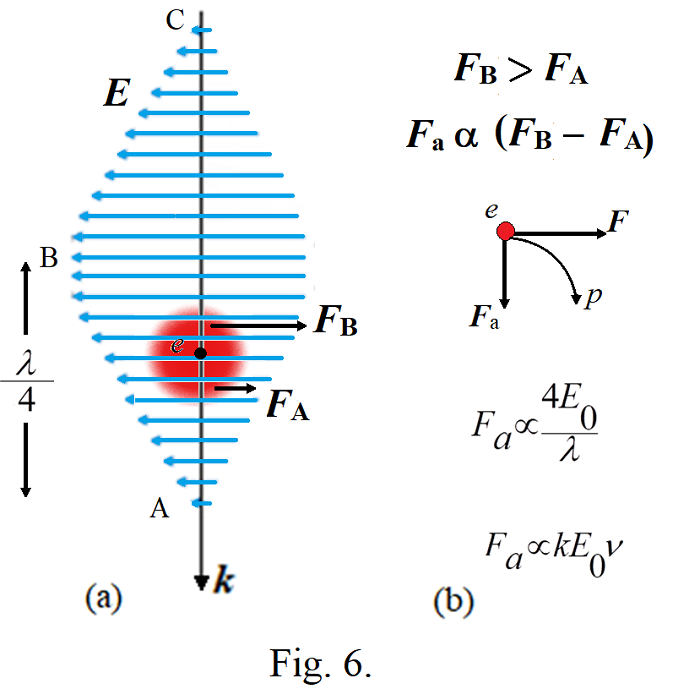We are investigating motion of the subjected electron when it is in the region between ‘A’ and ‘B’ of the electric field of the wave. Here we divide the electric field of the electron into two hemispheres, upper and lower. Let F be the net electric force divided into two parts FA and FB where FA is the force on the electron’s field at its lower hemisphere and FB is the force on the electron’s field at upper hemisphere. Clearly FA<FB because of which the net electric force F is asymmetric causing the electron to follow the curved path ‘p’. As FA<FB, the electron gets pushed into the metal surface and there no ejection from the metal. As the electron follows a curved path, therefore, it can be imagined as acting under two forces, the net electric force F which is always in opposite direction of the electric field of the wave and other is an apparent force Fa which is proportional to the electric field strength E0 of the wave and inversely proportional to the distance between points ‘A’ and ‘B’.Further it is always perpendicular to the velocity of the electron and it is in the plane of the electric field of the wave.Actually the apparent force can be true face of magnetic force arising due to the symmetric electric field and asymmetric electric force.

Thus,Thus the apparent force Fa is proportional to the frequency of the wave.

While progressing the wave, when the subjected electron at the metal surface comes at position ‘B’ of the wave, the electric force becomes symmetric and there is no curved path to be followed by the electron.

When the subjected electron comes at the position between point ‘B’ and ‘C’ of the wave, once again the electric force becomes asymmetric as shown in fig. 7.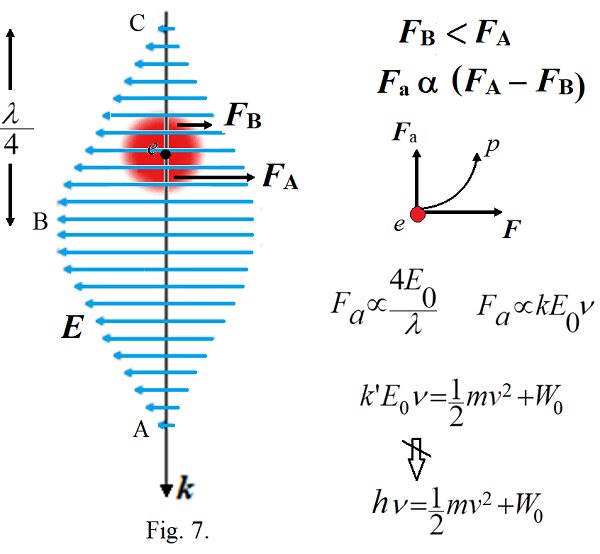Here FA>FB because of which the electron follows curved path in upward direction and may be ejected from the metal surface. Here again the apparent force, responsible to produce the curved path, is proportional to the frequency of the wave. The work done by this force in pushing the electron at the metal surface in upward direction may be assigned as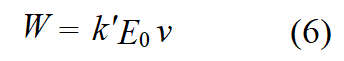Part of this work done will be used to overcome binding energy to release the electron from the metal surface and remaining will be converted into kinetic energy of the electron. If W0 is the binding energy of the electron then we may have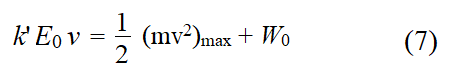This equation is similar to the Einstein’s equation (3) except k’E0 should be equal to the plank’s constant h which seems to be impossible.In Einstein’s explanation, one photon is absorbed at one time by an electron. According to this consideration, if one wave is linked to the subjected electron at one time then the amplitude E0 of the wave should be always constant, since it is emitted by transition of single electron in atom possessing fixed charge and field. Therefore, k’E0 should be always constant for all frequencies of the light waves. More investigation in this regard is needed. But at least equation (7) relates kinetic energy of the photoelectron with frequency of the incident light wave.

Thus we have gone step by step and point to point what should be happening at the position of the electron in photoelectric effect and found that the asymmetric electric field in the wave is responsible to produce the asymmetric electric force. The asymmetry in the electric force increases with increase in frequency of the wave because of which the kinetic energy of the photoelectron is found to be proportional to the frequency of the wave. This is the fact and is the last option in classical electrodynamics to relate the kinetic energy of the photoelectron with frequency of the incident wave.

#### Experimental proof: Increase of Magnetic Force with increase in Frequency of EM Waves

Asymmetric electric field produces asymmetric electric force which is responsible to produce the apparent force which is always perpendicular to the velocity of the subjected charged particle and it exists only when the charged particle is in motion. Therefore, it creates possibility of the apparent force can be the true face of magnetic force. If it is the fact then it could be experimentally verified. As the apparent force increases with increase in frequency of light wave causing to produce the photoelectric effect and the apparent force if is the true face of magnetic force then, the magnetic force existed by any electromagnetic wave like produced by an antenna should increase with increase in frequency of the wave which could be experimentally verified as explained below.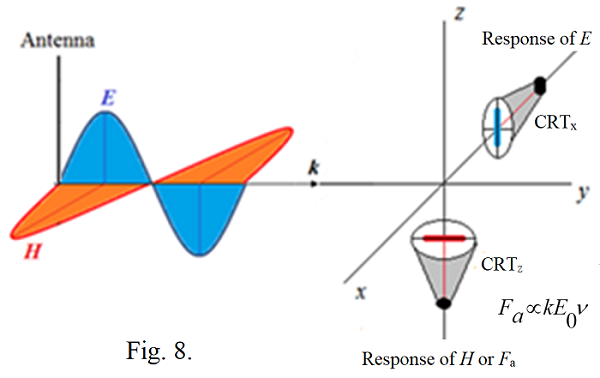Fig. 8 represents the experimental arrangement to detect the dependence of magnetic force on frequency of the EM wave and phase difference between electric and magnetic fields in the wave. The antenna produces EM wave of suitable frequency and amplitude. Two cathode ray tubes (CRTs) can be used to detect response of the electric field and magnetic field in the wave. CRTx is kept perpendicular to the electric field to detect its field strength. CRTz is kept perpendicular to the magnetic field to detect its field strength.

Deflection of CRTx will be proportional to the amplitude of the electric field wave. This deflection will not depend on frequency of the wave. According to the classical electrodynamics, there will be no effect of magnetic field wave on deflection of CRTx as its electron beam is parallel to the magnetic field. By increasing the frequency of the EM waves with keeping amplitude of the wave constant, we should find there is no change in the deflection of the electron beam of CRTx.

Deflection of CRTz will be proportional to the amplitude of the magnetic field wave. According to the classical electrodynamics,this deflection will not depend on frequency of the wave, since the magnetic force equation is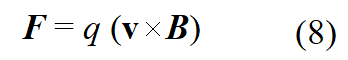It does not involve the frequency of the wave.

Further, there should be no effect of electric field wave on deflection of CRTz as its electron beam is parallel to the electric field. By increasing the frequency of the EM waves with keeping amplitude of the wave constant, we should find no change in the deflection of the electron beam of CRTz also.

But if the apparent force is actually working as the magnetic force, then with increase in frequency of the wave the deflection of the CRTz should increase though the field strength of the wave is constant. It can be explained by fig. 9.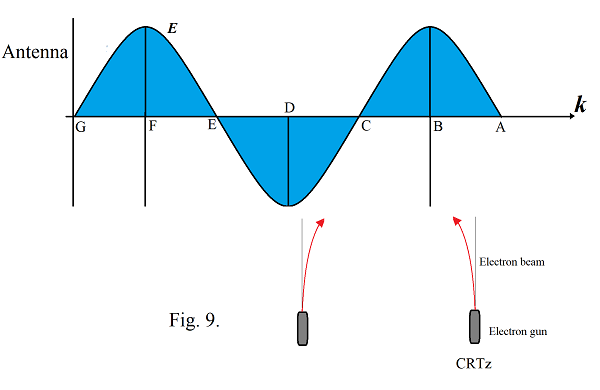If the apparent force is the true face of magnetic force then the magnetic field is absent in the wave. From fig. 9, the electric field in the region A to C tries to decelerate the electron beam because of which the electron beam gets deflected into the strong field region. This deflection is proportional to the amplitude E0 of the wave and inversely proportional to the distance between points A and B which is λ/4 consequently the deflection is proportional to the frequency of the wave with E0 is kept constant. The electric field in the region between point C and D tries to accelerate the electron beam because of which the beam gets deflected into the weak field region. Here also the deflection is proportional to the frequency of the wave. This experiment will prove whether the apparent force is working as the true face of magnetic field or not.

This experiment can be performed such as suppose the length of the electron beam of the CRT is 10cm and the accelerating voltage of the final anode is 1000volt then the frequency of the EM wave should be in the range of 1MHz to 100MHz. Produce such an EM wave by an antenna and keep the magnitude of the wave sufficiently strong so as to get deflection of electron beam in CRTs. By keeping the field strength constant and increasing the frequency of the wave from 1MHz to 100MHz in small steps and note the deflections of the CRTs at both positions. With increase in frequency we should found increase in deflection of the CRTz which will confirm the apparent force Fa produced by asymmetric electric field which is working as the magnetic force and responsible to produce the photoelectric effect. On the other hand CRTx should not show any change in the deflection. While doing the experiment we might have to do changes in the frequency range.

#### Phase difference between electric and magnetic field waves:

If the apparent force is actually working as the magnetic force then there can be a phase difference between electric field wave and magnetic field wave which can be understood using figure 10. When electrons are accelerated, they get pushed into weak field and when they are decelerated then they get pushed into strong electric field.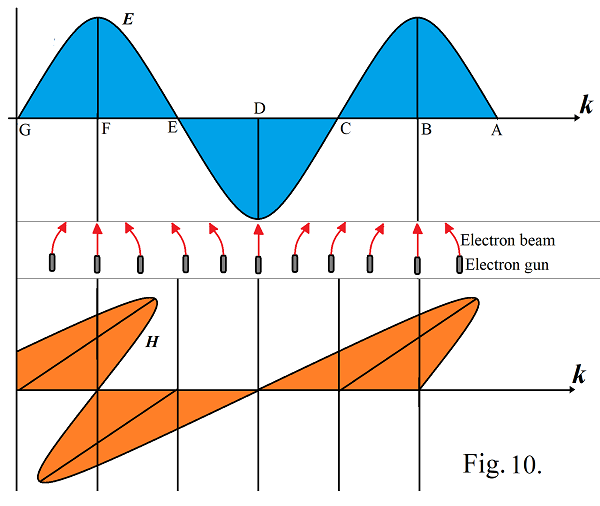The electric field between A to C decelerates the electron because of which they get pushed into the strong field region. The field between C to E accelerates the electron because of which they gets pushed into the weak field region.

At positions B, D, F the electric field is symmetric, therefore, there is no deflection of electron beam indicating corresponding magnetic field should be zero. The phase difference can be experimentally verified by using same ramp voltage signal to both CRTs simultaneously. The actual phase difference between electric field and magnetic field in an EM wave should be as like shown in figure 11 (b).Fig. 11 (a) represents no phase difference between electric and magnetic field waves in according to the existing classical theory of electrodynamics.

Fig 10(b) represents the expected phase difference between electric and magnetic field waves from the above discussion.

#### Conclusions:

1. In EM waves, the magnetic force should increase with increase in frequency which should be responsible to produce the photoelectric effect.

2. There should be a phase difference of 90 degree between electric field and magnetic field in EM waves as described.

3. The apparent force produced due to the asymmetric electric force is the true face of the magnetic force, which gives answer to the absentee of magnetic monopoles in the universe.

#### References:

1. G. H. Jadhav, ‘Photoelectric Effect: Magnetic Force should increase with increase in Frequency of EM Waves’ Presented in ICAMSER-2021 and accepted for publication in AIP proceeding, 2021.

## On estimation of total length of single light wave emitted by Sodium atom using air-wedge method

While dealing with wave nature of light, we know its wavelength, frequency and speed.We don’t know yet about the total length of the light wave at the instant when it is emitted by an atom. The time taken by an electron for transition from its higher energy level to lower energy level in an atom should be finite.Therefore, the total length ‘L’ of the light wave emitted in this process should be finite.However, it could be how much long for a particular transition of the electron is not estimated yet.The Newton’s ring experiment is generally performed to determine average wavelength of sodium light. Typical students are always interested to know how many rings are formed there just because of their curiosity. They face difficulty due to decreasing width of the rings going away from the center where the colour gets diffused more and more. How many rings should be formed there, in fact, gives important message about the total length of the single light wave involved there and is being ignored always.

However, instead of using Newton’s ring experiment, we can use the air-wedge method where the fringe width remains constant all over which ease to estimate how many fringes are being formed for a given monochromatic light source. However as the path difference increases the fringe colour gets diffused and diffused and lastly becomes difficult to discriminate bright and dark fringes. Therefore, we have approximately estimate the total number of fringes formed there.

#### Number of lines emitted by sodium atom:

Here we describe how one can estimate the total length of single light wave emitted by a sodium atom using simple air-wedge method.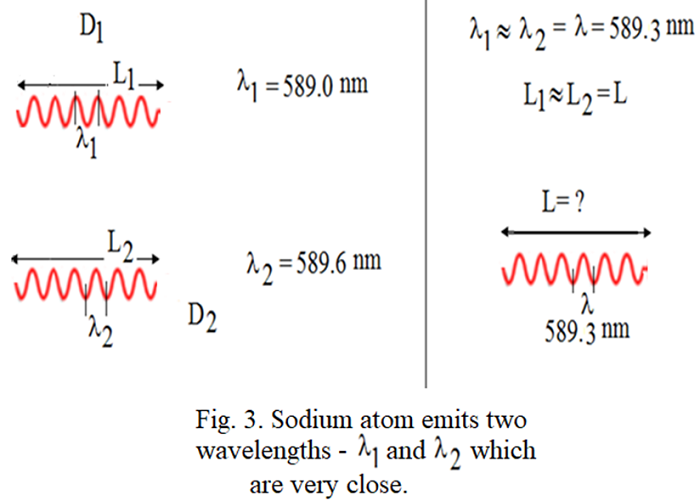#### Air-wedge method to determine total length of single wave emitted by sodium atom:

Air-wedge method is generally used to measure thickness of a thin wire using a monochromatic light of known wavelength. Our interest is to measure total number of fringes formed there . Corresponding experimental arrangement is shown in fig.1. Instead of a thin wire placed between glass plates G2 and G3, a cover slip usually, used in biology lab, may be placed to obtain suitable air-wedge producing distinguishable fringes to count without difficulty. A sodium lamp is used as a monochromatic light source. Actually, sodium light has two wavelengths with extremely close to each other as described in fig.2. The air-wedge method cannot discriminate such small difference in wavelength and treats as waves of one average wavelength is 589.3nm Further, we expect that, once a wave, either having wavelength 589.0nm or 589.6nmemitted by a sodium atom should have a finite total length as shown in fig. 2. Our attempt is to find the total average length L in vacuum, with assuming that     L1 = L2 = L, of single wave of sodium light. For that, we go systematically in following way.

No. of waves are emitted simultaneously by sodium atoms constituting the sodium lamp.Each wave has finite total length L. With reference to figure1, every single wave A emitted from sodium light source has total length L. Every wave A is partially reflected and refracted at glass plate G1.The corresponding partially reflected wave B has same total length L.Further every wave B is responsible to produce corresponding wave 1 reflected at lower surface of glass plate G2 and wave 2 reflected at upper surface of glass plate G3. Therefore, wave 1 and wave 2 have same total length L.Every wave 1 and corresponding wave 2 contributes to the formation of dark and bright fringes.The light wave 2 reflected from lower surface of glass plate G2 has no phase shift. The light wave 1 transmitted into the air travels a distance, t, before it is reflected at the upper surface of glass plate G3.Reflection at the air-glass boundary causes a half-cycle (180°) phase shift because the air has a lower refractive index than the glass. The reflected light at the lower surface returns a distance of (again) t and passes back into the lens. Thus the net or effective path difference between wave 1 and wave 2 is the actual path difference plus λ/2 or2t+( λ/2).At contact point P1 of glass plates G2 and G32t=0, therefore, the effective path difference is λ/2. Consequently the waves interfere destructively producing first dark fringe as shown in figure 4.Going from point P1 to point P2the actual path difference between wave 1 and wave 2 increases and becomes 2t=λ/2, the waves come in phase with each other and form the first bright fringe. Here wave 1 lags behind the wave 2 by distance λ/2.Further to right of that, the path difference between the two waves increases and becomes 2t=λ=, where the two waves, once again, come out of phase with each other and form second dark fringe.In this way, when we go from point P1 to point P2 the path difference between wave 1 and wave 2 increases forming dark and bright fringes alternatively. A point will reach at which the path difference between the two waves becomes 2t=L where there is no formation of fringes as the two waves become separate.While counting the fringes, the telescope is scanned along x-axis and the lamp is also kept on the same axis so that the beam intensity is maintained.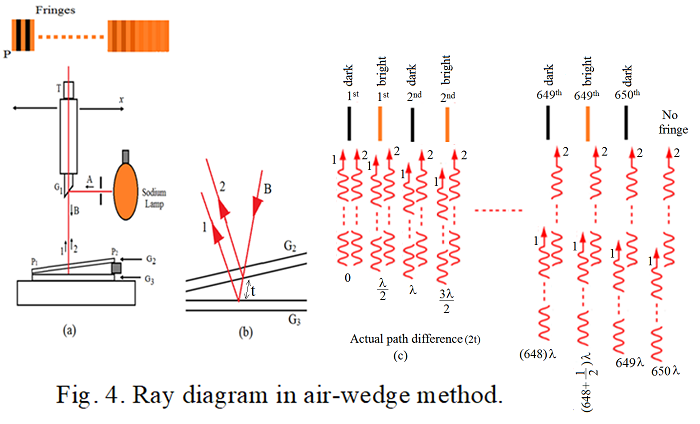Counting total number of dark fringes multiplied by wavelength of the wave gives the total length of wave 1 or of wave 2 which is equal to the total length of wave A. However, the overlap portion of wave 1 and wave 2 reduces as the path difference between them increases. Therefore, practically it becomes difficult to estimate exact total number of bright and dark fringes formed in this system, especially in the region where the wave 1 and wave 2 are close to become separate. However, approximate estimation of total number of fringes can be done which gives the approximate estimation of total length L of the wave A. We could thus approximately estimate formation of 650 number of dark fringes for sodium lamp by using air-wedge method in undergraduate physics laboratory.

#### Results:

Total number of dark fringes formed are n, then the total length of the monochromatic single light wave is L=nλ. Here total number of dark fringes formed are 650. Therefore, total length is,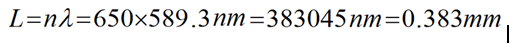The total length of the light wave emitted by sodium atom is 0.383mm and is very long. How such long wave can act as a single photon how it can be absorbed by a single electron is amazing. Since electron size is simply 0.1nm.

#### Combination of two waves to form a single wave:

At first glance one cannot expect that a sodium atom is emitting wave having total length approximately equal to 0.383nm. It means that there can be possibility of two or more waves that are joined together to form a chain of waves consequently the actual wave emitted by the sodium atom and the wave A, who is taking part to form the fringes, may be different from each other. This can happen as there are number of sodium atoms enclosed and distributed in the sodium lamp which are emitting light waves simultaneously. To check this possibility, we consider combination of two waves in different ways to form a chain of wave as illustrated in figure 5.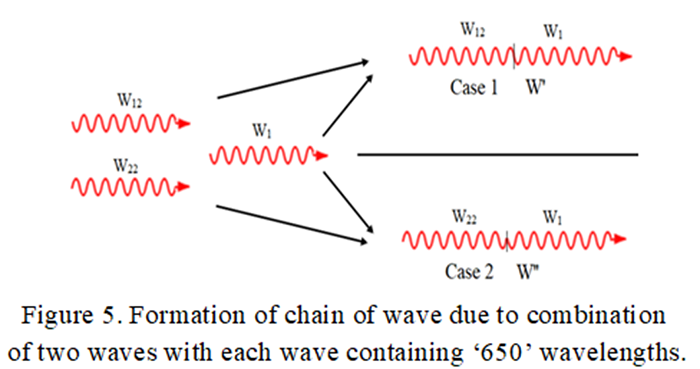Wave W1 and W12 emitted by two different atoms are joined in their journey such that they form a single wave W as illustrated there. Suppose there are 650 number of wavelengths in waves W1 (same in waves W2 too) then 650 number of dark fringes are formed by this wave. Further, at the place of 650th fringe (path difference 2t=(650+(1/2))λ)), the wave W' forms a bright fringe as shown by case 1 in figure 6(a).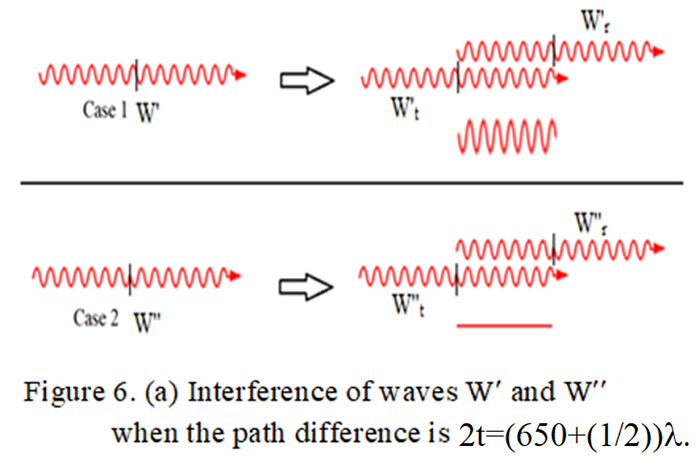At the same time the resultant wave W’’ forms a dark fringe at the same place as shown by case 2. For further path difference of λ/2 they will interfere such as illustrated in figure 6(b). At this place the wave W' forms a dark fringe whereas the wave W''forms a bright fringe. Thus, in the further region only dark or bright fringes cannot be formed. We have taken two typical cases of waves W' and W'' for examination. It is true for all possible combinations of the waves emitted by the sodium lamp. Hence, the total length, 0.383mm, of the wave A obtained in this experiment should be the actual total length of the wave emitted by the sodium atom in the lamp.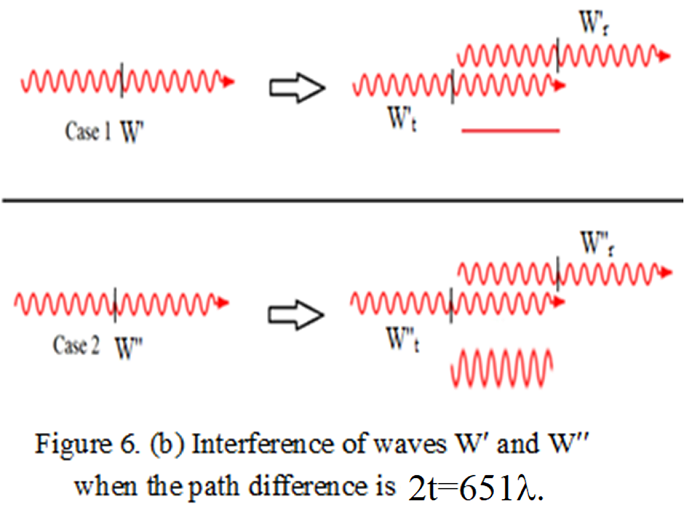#### Discussion:

According to the classical electrodynamics, one oscillation of electron should emit wave containing one wavelength. Two oscillations of electron should emit wave containing two wavelengths. Therefore, if a wave containing 650 wavelengths, then the corresponding electron should be oscillated 650 times while producing the wave.It is amazing. Therefore, the light emission mechanism in atoms is yet to be understand properly.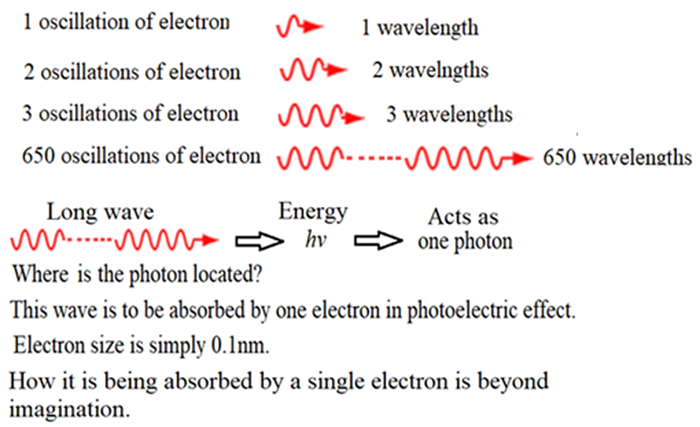Further, the total length of a wave can depend on the size of the emitting atom, temperature of substance, state of the substance-gas or liquid or solid etc.Different monochromatic light sources can be used to find the total length of their light waves. From any light source, a monochromatic light wave can be also isolated to determine its total wavelength.Such data may give new information about the light emitting mechanism of the atoms.

#### Conclusion:

The total length of single light wave emitted by a sodium atom is 383045nm approximately and is very long.

#### Reference:

1. G. H. Jadhav and ShivanandKalshetty, ‘On estimation of total length of sodium light wave using air-wedge method’, Presented in ICAES2021 and accepted for publication in AIP proceeding, 2021.

## What is magnetic field?

#### Conclusions from the photoelectric effect:

1. In EM waves, the magnetic force should increase with increase in frequency which should be responsible to produce the photoelectric effect.

2. There should be a phase difference of 90 degree between electric field wave and magnetic field wave in EM waves.

3. Both things are not supported by classical electrodynamics.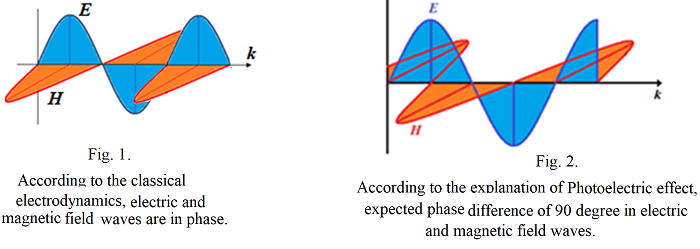#### Fields and their sources: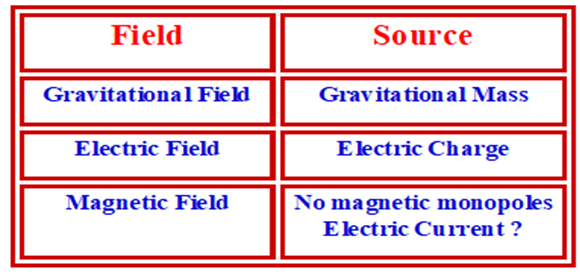Magnetic monopoles are not found in the universe. They cannot be created in laboratories.
In absence of magnetic monopoles, magnetic field is created, why?
Some interactions created due to electric charges are to be explained in terms of magnetic fields and magnetic forces instead of electric fields and electric forces. That is why we expect something is there that yet to understand.
Our attempt is to trace what could be that.

#### Complications in Magnetic field and Magnetic force:

What do we expect from a field?
Field should be just like a pressure in the surrounding region of the substance. For electric current, it should be either in the direction of the current or opposite.
Force applied by the field on any competent particle should be either in the direction of the field or in opposite.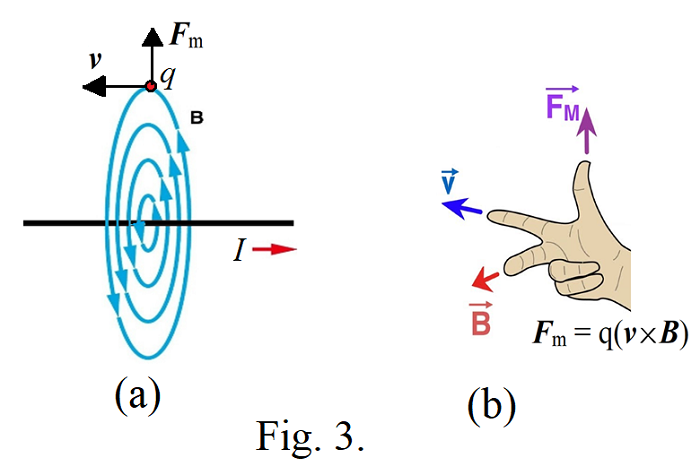But according to the classical electrodynamics the field produced by a straight current is circular as shown in fig. 3(a). Further the force is not in the direction of the field or opposite. It is perpendicular to both the direction of the field and velocity of the particle.
So things are complex.

#### Reinvestigating the field of a current carrying conductor:

It would be a simple approach to investigate what type of field is being produced by a straight long conductor carrying steady electric current . For that we use a cathode ray tube.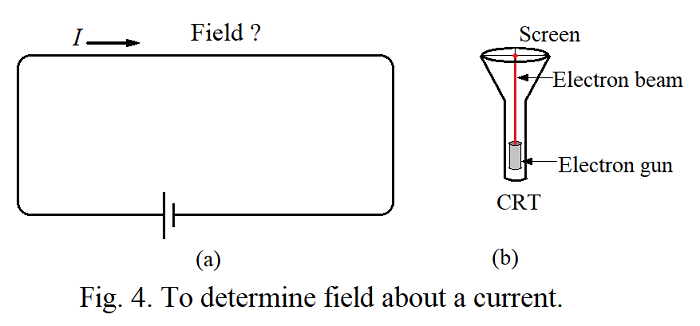#### Assumptions:

Conductor contains electric charges and no magnetic cahrges. Electric field around the conductor is null before carrying the electric current. Flow of steady electric current throught the concutor causes to move only negative electric charges in one direction. Therefore, the electric field around the conductor cannot be null now. Electric field is simply a pressure around the conductor and can be experienced by placing electric charges in it. This field should apply force on the charged particle in one directiononly. Aim is to find the structure of the field.

Deflections of electron beam near straight long conductor carrying steady electric current are shown in fig. 5. At first glance it seems that the electron beam in different directions is experincing forces in different directions which is unexpected. Force should be always in one direction. Therefore, we have to anylayse one by one.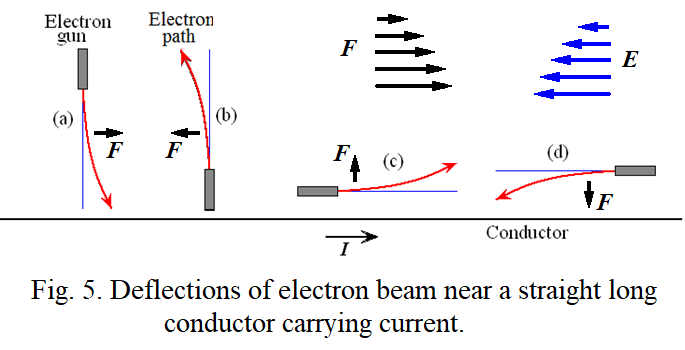Clearly, when we consider the case (a), the force F should decrease when we go away from the current as shown in fig. 6. Further, if it is produced by electric field E then the field should be in opposite direction of the force as shown in fig. 6. The force experienced by the electron should be asymmetric in nature and should be existed in terms of field-field interactions as explained below.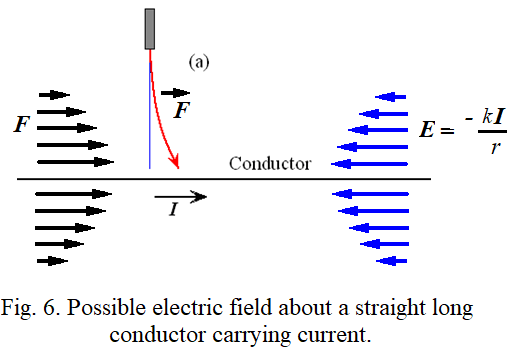#### Force through field-field interaction:

Let us see generalization of the electric force with time in classical electrodynamics.
Case (1):In fig. 7(a), the force between the two charges is expressed in terms of their charges. It may be considered as force in terms of charge-charge interaction.Case (2): In fig 7(b), charge q1 produces electric field E1 around itself. If another charge q2 is brought into the field then the field applies force on the charge. It may be considered as force in terms of field-charge interaction. The force equation is given by F2 = q2E1, where E1 is the electric field produced by charge q1 at position of charge q2. Force is mutual. Therefore, charge q2 also applies force on charge q1 in terms of field-charge interaction by F1 = q1E2, where E2 is the electric field produced by the charge q2 at position of charge q1.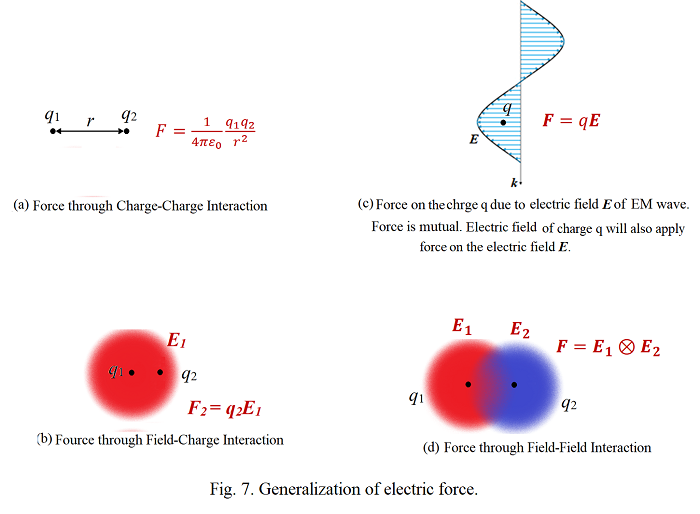Case (3): In fig. 7(c), an electric field wave in an electromagnetic wave is propagating with speed of light and interacts with a charge q at an instant. This field also applies force on the charge in terms of field-charge interaction. If E is the electric field of the electric wave at position of the charge q at an instant then the force equation is F = qE . But the force is mutual. Hence the charge q should also apply force on the electric field of the wave. As there is no charge associated with electric field of the wave, the electric field of the charge q must apply force on the electric field of the wave which may be considered as force through field-field interactions. Obviously, as the subjected charge q is always associated with its own electric field, therefore, the electric field of the applied wave must apply force on the field of the charge resulting into the force through field-field interactions. Thus finally one can conclude that the electric force exists not in terms of charge-charge interaction or field-charge interaction but it exists in terms of field-field interactions.
Case (4): In fig. 7(d), if two charges are brought close to each other, then force between them, either attractive or repulsive, is existed in terms of their field-field interactions. It means the electric field of first charge exerts force on the electric field of the second charge and vice versa. Thus a new electric force equation is to be developed which could explain the force in terms of the field-field interaction. Thus, we consider existence of electric force in terms of the field-field interactions for further discussion.
Using the concept of force through field-field interactions, let us once again explain the deflections in fig. 5 with reconsidering in fig. 8.
In case (a) of fig 8, the front field of the electron in the electron beam experiences greater force than the back field because of which it gets pushed along the direction of the force. In case (b), the back field of the electron experiences greater force than the front field because of which it gets pushed in the opposite direction of the force. In case (c), the lower field of the electron in the electron beam experiences greater force than the upper field while acceleration because of which it gets pushed into the weak field region. In case (d), the lower field of the electron experiences greater force than the upper field while deceleration because of which it gets pushed into the strong field region.
Things seem to be very different from our belief. But according to the discussion of photoelectric effect, if we found increase in frequency of EM wave causes to increase in magnetic force and a phase difference of 90 degree in electric field wave and magnetic field wave in EM wave, then we are compelled to reinvestigate the real nature of magnetic field. At present, we have no any other option than to accept the above possibilities.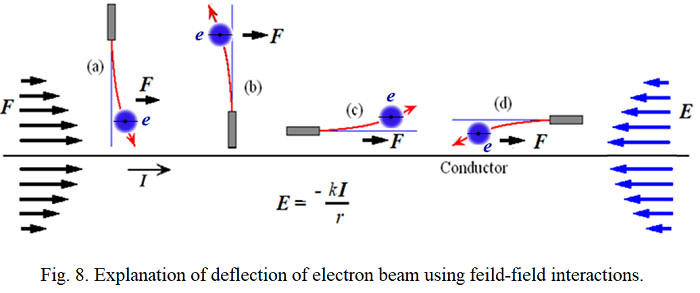#### Electric field of coil and bar magnet:

Electric field is parallel to the current and in opposite direction. Therefore a circular current carrying coil should a circular electric field.Electric field near the conductor of the coil is maximum. Therefore, cones of electric field are formed at both ends of the coil. Surface of the cone is formed where the magnetic field lines get turned. Electric field is maximum at the surface of the cone. Electric field decreases away from the coil.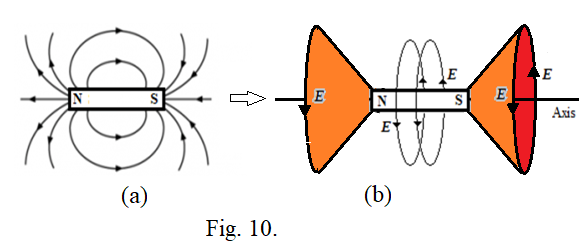In bar magnet, spin of unpaired electrons is aligned in such a way to produce non-zero effective current by them. This current is responsible to produce a circular electric field around the bar magnet as shown in fig. 10(b). Obviously, this electric field is in opposite direction of the effective non-zero current. Two cones of electric field are formed at both said poles of the magnet.Electric field is maximum on surface of the cones and decreases away from the bar magnet in all directions.
Current produced in a superconductor supports to the circular electric fields produced by a current carrying coil and a bar magnet.
The electric field of the coil tries to accelerate the charged particles in the direction of the field producing circular current in the superconductor because of which the superconductor gets pushed away from the coil in the weak field. Because of that we consider superconductor as a diamagnetic material.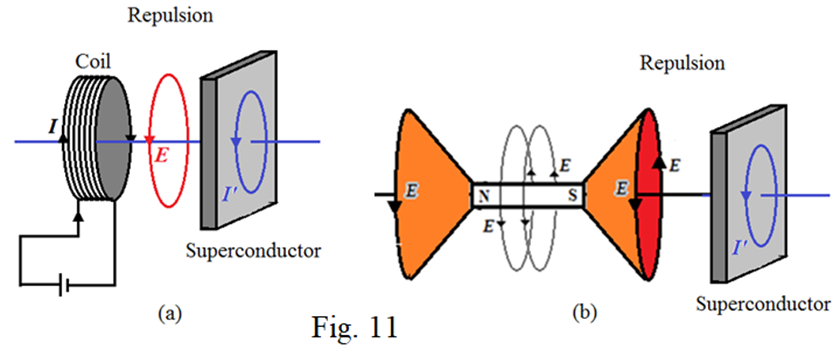Similarly the electric field produced by the current in the superconductor pushes the coil apart resulting into the repulsion between them because of which the superconductor is considered as diamagnetic material. Similar thing happen with bar magnet.

Such current is not produced in ordinary conductors and is a part of investigation. If the applied electric field is alternating then produces alternating current in ordinary conductor and is governed by Faraday’s law of electromagnetic induction. Though we know magnetic field and magnetic force, why we require the Faraday's law to tell emf produced in secondary circuit is really beyond our imagination.

#### Verification of the electric field cones produce by circular current:

As the electric field is maximum close to the current, therefore, electric field strength should be maximum near the wires forming circular cones at both ends of the coil. The electric field in the region of the cone should increase away from the axis of the coil towards the surface of the cone while going in perpendicular to the axis. It should be maximum on the surface of the cone. Similar type electric field should also be produced by a bar magnet. The direction of the electric field is same everywhere in the surrounding region of the coil and bar magnet and is circular about axis of the coil.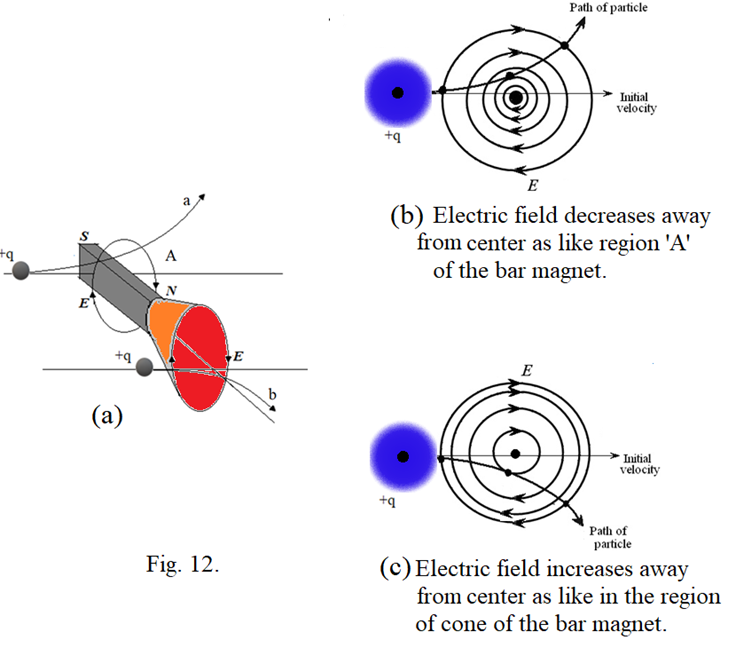The deflections of a charged particle in the electric field of bar magnet are shown in fig. 12. In region ‘A’ of the bar magnet it goes in upward direction while in the region of cones it goes in downward direction thought the field direction is same at both regions. This is because in which direction the field is increasing or decreasing means the field strength is increasing or decreasing while going away from its center will decide in which direction the particle is to be deflected. In region ‘A’ of the magnet, the electric field decreases away from center of the bar magnet which is illustrated in fig. 12(b). The charged particle deflections can be understood using the four deflections shown in fig. 8. If the particle is coming into the strong field region then it gets deflected in the direction of the force. If the particle is going into the weak field region then it gets pushed in opposite direction of the force. If the particle gets accelerated then it gets pushed into the weak field region and if it gets decelerated then it gets pushed into the strong field region. Using these facts, the deflections in the surrounding region of the bar magnet can be understood which supports to the formation of the electric field cones. Possible electric field cones at both poles of earth are shown in fug. 13. Formation of aurora in ring shape at both poles of the planets (fig. 14) supports to the formation of electric field in the form cones at both poles of the planets.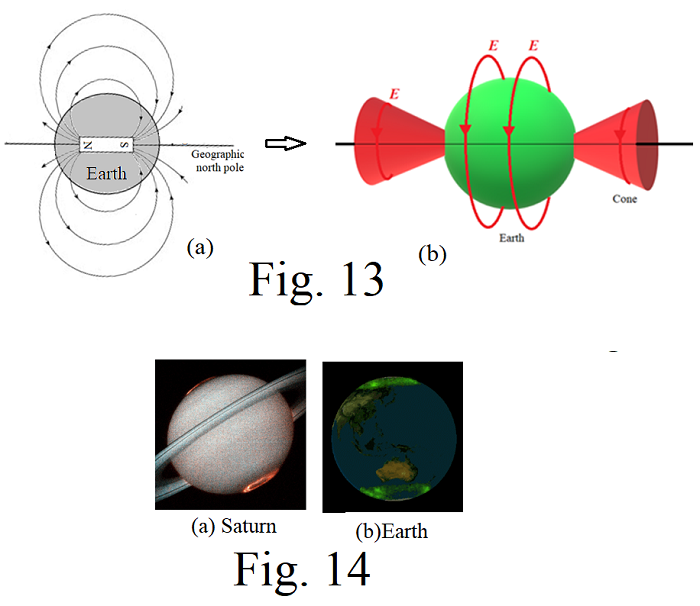#### Attraction between poles of magnets:

Attraction and repulsion between magnetic poles is an important phenomenon in magnetism. In fig 15(a), the electric force and spin direction of the electron are opposite to each other. Therefore, the electron gets dragged towards the magnet into strong electric field. In fig. 15(b), the electric field of magnet M1 applies force on the unpaired electrons in magnet M2 in opposite direction of their spin because of which these electrons and hence the magnet M2 gets dragged towards magnet M1. In similar way the electric field of magnet M2 reacts with the unpaired electrons in magnet M1. Thus there is no direct attraction between the magnets.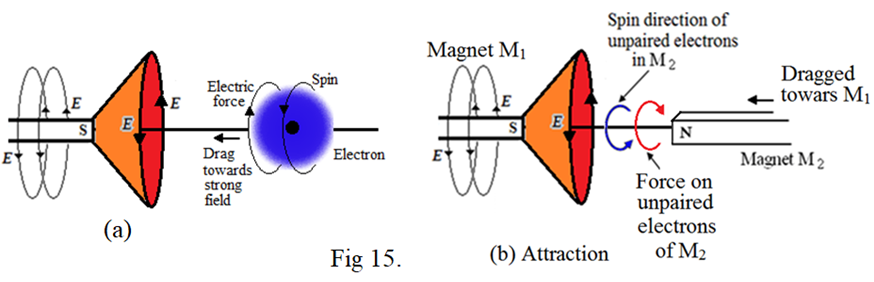#### Repulsion between poles of magnets:

In fig 16(a), the electric force and spin direction of the electron are in same direction. Therefore, the electron gets pushed away from the magnet into weak electric field.
In fig. 16(b), the electric field of magnet M1 applies force on the unpaired electrons in magnet M2 in same direction of their spin because of which these electrons and hence the magnet M2 gets pushed away from magnet M1. In similar way the electric field of magnet M2 reacts with the unpaired electrons in magnet M1. Thus there is no direct repulsion between the magnets.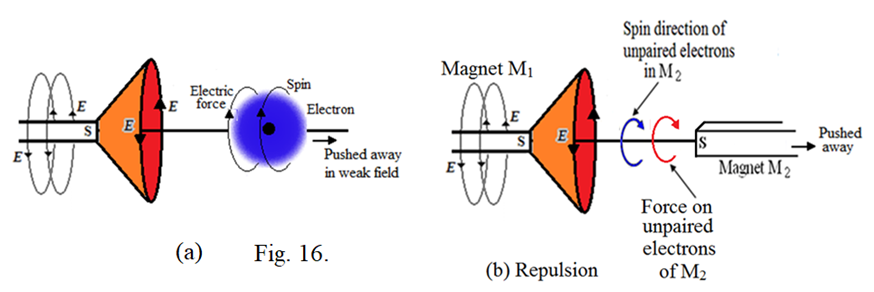#### Magnetization produced in paramagnetic or ferromagnetic materials:

Magnetization produce in magnetic materials is an important phenomenon in magnetism.
Suppose a paramagnetic or ferromagnetic material is brought near a bar magnet. If an unpaired electron in the material possesses spin in opposite direction of the force applied by the electric field of the magnet then there will be attraction between the electron and the magnet resulting no change in the spin direction. There will be no change in the spin direction of all such electrons.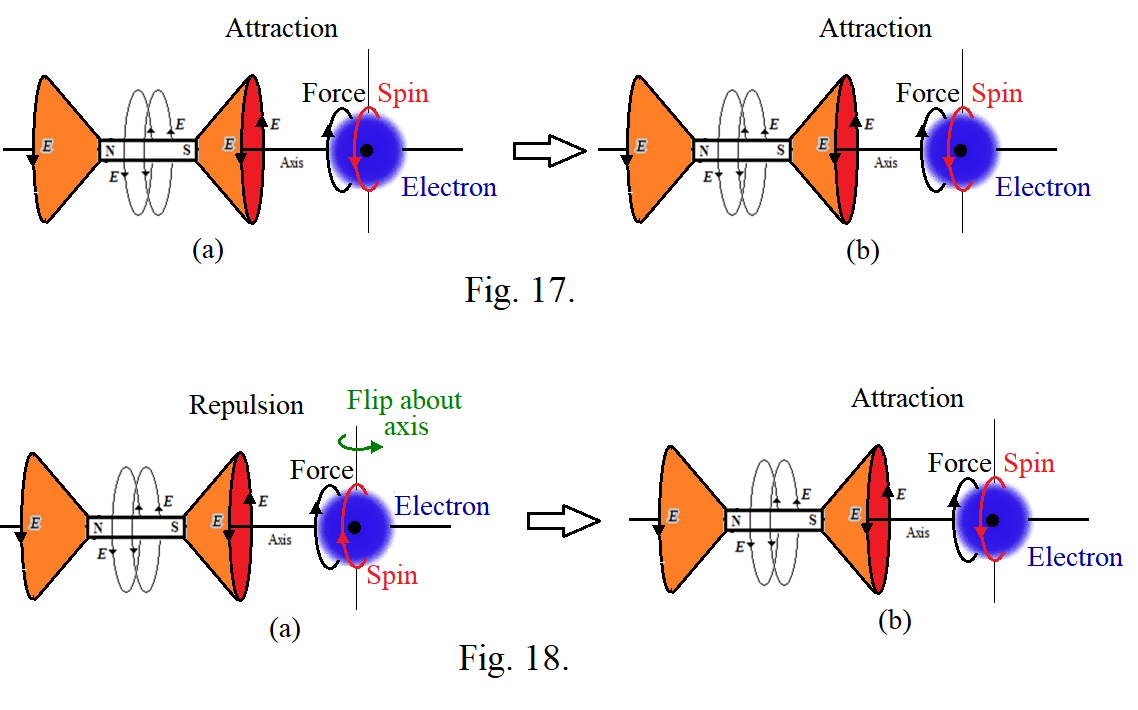If an unpaired electron in the material possesses spin in same direction of the force applied by the electric field of the external magnet then there will be repulsion between the electron and the magnet resulting flip of the spin of the electron about the vertical axis as shown in fig. 18. It tends to align the spin of the electron in opposite direction of the force producing attraction between them and no flip of the spin further. It happens for all such electrons. In this way the spin of all unpaired electrons get aligned in opposite direction of the force produced by electric field of external magnet. Such alignment of the spin of unpaired electrons in the paramagnetic or ferromagnetic material is nothing but the magnetization. Due to the spin alignment of the unpaired electrons in the material, its net electric field becomes nonzero and it will be circular and will be in the same direction of the spin motion of these electrons or in the same direction of the electric field of the external magnet.

#### Conclusion:

According to the discussions in the photoelectric effect, the magnetic force should increase with increase in frequency of electromagnetic wave. Further, there should be a phase difference of 90 degrees between the electric field wave and magnetic field wave in an EM wave. Both things are not supported by the classical electrodynamics. Further it is found that the apparent force produced due to the existence of asymmetric electric force works similar to the magnetic force implying that the apparent force can be the true face of magnetic force giving answer to the absentee of magnetic monopoles in the universe. On advent of these findings, a straight current should produce a parallel electric field and a coil and a bar magnet should produce a circular electric fields with having two electric field cones at the said poles of the magnet. Related things should go as discussed above. Further investigation is essential in this regard.

#### References:

1. G. H. Jadhav, On static curled electric field produced by a steady current of magnetic monopoles, Proceeding of ‘2011 Annual Meeting of Electrostatic Society of America’, pp.1-7 (2011).

## Spin Atomic Structure

#### Introduction:

Up to the 19thcentury, atoms were believed to be the smallest units of matter that are indivisible.The first breakthrough came in the late 1800s when English physicist Joseph John Thomson discovered that the atom wasn’t as indivisible as previously claimed.He carried out experiments using cathode rays produced in a discharge tube, and found that the rays were attracted by positively charged metal plates but repelled by negatively charged ones. From this he deduced the rays must be negatively charged. Thus, in 1897, he discovered the electron. He believed atoms could be divided. Because the electron carried a negative charge, he proposed a plum pudding model of the atom in 1904, in which electrons were embedded in a mass of positive charge to yield an electrically neutral atom.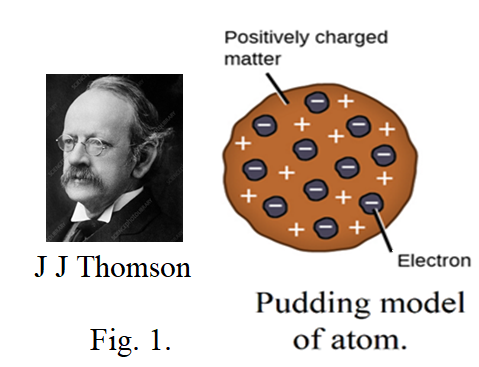Ernest Rutherford was a physicist from New Zealand who studied at Cambridge University under Thomson. It was his later work at the University of Manchester which would provide further insights into the insides of an atom. He devised an experiment to probe atomic structure which involved firing positively charged alpha particles at a thin sheet of gold foil and found that the positive charge of an atom and most of its mass were at the center, or nucleus of an atom. Therefore, he proposed a planetary model in which electrons orbited a small, positive-charged nucleus.Rutherford was on the right track, but his model couldn't explain the emission and absorption spectra of atoms, nor why the electrons didn't crash into the nucleus.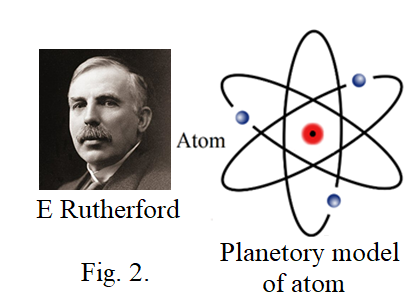In 1913, Niels Bohr proposed the Bohr model, which states that electrons only orbit the nucleus at specific distances from the nucleus. According to his model, electrons couldn't spiral into the nucleus but could make quantum leaps between energy levels. He postulated the existence of energy levels or shells of electrons. Electrons could only be found in these specific energy levels; in other words, their energy was quantized, and couldn’t take just any value. Electrons could move between these energy levels referred as ‘stationary states’ but had to do so by either absorbing or emitting energy.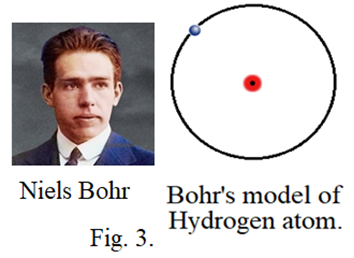Bohr’s model didn’t solve all the atomic model problems. It worked well for hydrogen atoms, but couldn’t explain observations of heavier elements. It also violates the Heisenberg Uncertainty Principle. Thus the Bohr’s model was still required refining. At this point, many scientists were investigating and trying to develop the quantum model of the atom.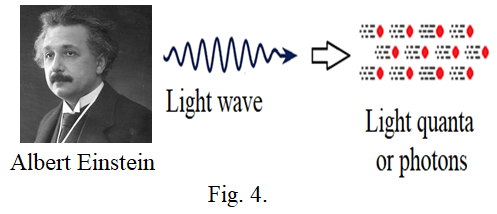While solving the crisis of photoelectric effect, Einstein had published his paper in 1905 providing revolutionary explanation on the photoelectric effect. It was based on his “light quanta” hypothesis. He proposed that the light behaves as a stream of independent, localized units of energy called ‘light quanta’ each light quanta has an energy hv where v is the frequency of the light wave and h is the plank’s constant. As light waves can behave as light quanta like particles, then a particle should also behave as a wave. This proposition was first put by L de Broglies proposing duel nature of matter waves.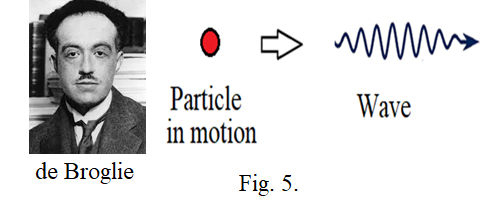That concept was further used by E Schrödinger. In 1926 E Schrödinger proposed that, rather than the electrons moving in fixed orbits or shells, the electrons behave as waves. Schrödinger solved a series of mathematical equations to come up with a model for the distributions of electrons in an atom. His model shows the nucleus surrounding by clouds of electron density. These clouds are probability of finding the electrons though we don’t know exactly where they are. These regions of space are referred to as electron orbitals.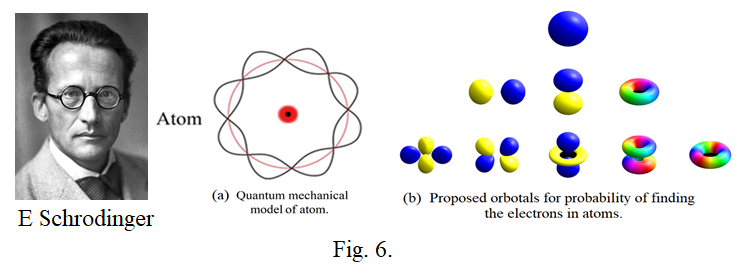In 1932, the English physicist James Chadwick discovered the existence of the neutron, completing our picture of the subatomic particles that make up an atom. The story doesn’t end there either; physicists have since discovered that the protons and neutrons that make up the nucleus are themselves divisible into particles called quarks.
Until the end of 20thcentury, there was no any definite idea orunderstanding of atom. Development of the atomic models made clearthe fact that the nucleus is at the central place of the atom and electrons are just distributed around the nucleus. But how the electrons can maintain their safe distance from the nucleus is still part of debate. Schrödinger proposed that the electrons behave as waves rather than moving in fixed orbits or shells. He developed mathematical model for distribution of electrons in atom showing the nucleus is surrounded by clouds of electron density. These clouds give the probability of finding the electrons and are referred to as electron orbitals. But the photoelectric effect explanation proposes that in electromagnetic waves, magnetic force increases with increase in frequencyindicating that the magnetic force in light wave might be responsible to produce the photoelectric effect. The discussion further suggests that the asymmetric electric force produced by electric field in the light wave is responsible to produce an apparent force which can be the true nature of magnetic force. If the magnetic force or the apparent force in light wave is found to be responsible to produce the photoelectric effect then the duel nature of matter is to reconsider once again. It may be suggesting that the electrons are not behaving as waves. On this advent, how the electrons in the atoms are being distributed around the nucleus becomes unanswered.Aim of this presentation is to make investigation in this regard.

#### Spin of electrons:

Electron spin refers to a quantum property of the electrons and we believe thatit is a fundamental property just like charge and rest mass.Therefore, the spin should be always associated with the electron though it is in atom or free from atom (Fig. 7.).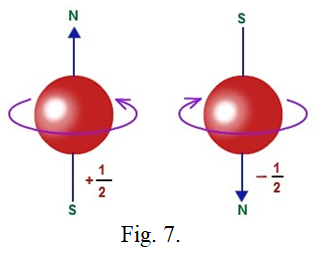Unfortunately we sometimes ignore on experiments, that are being performed in general physics laboratories, giving valuable scientific information. Such one experiment is e/m by Thomson method giving valuable information about spin of electron is intrinsic or not.A particular deflection of electron beam, in this experiment, due to the magnetic field between two poles of bar magnets is shown in figure 8.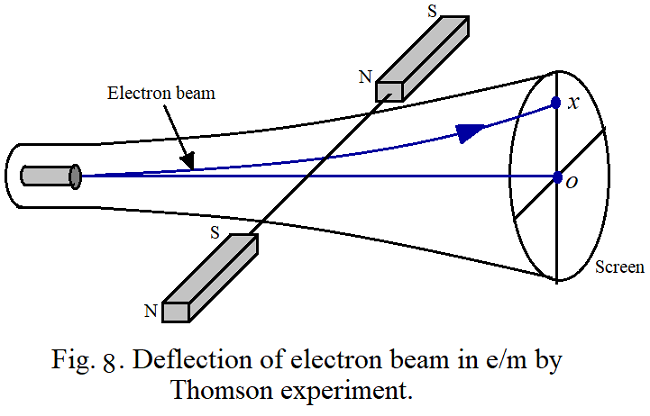Before application of the magnetic field, the electron beam was sharp. After application of the magnetic field, the electron beam is however deflected in upward direction but is again sharp producing a single spot on the screen. However, if electrons possess intrinsic spin, a line should be produced on the screen which is not found indicating that the electrons do not possess intrinsic spin which could be understood from the following explanation using asymmetric electric field and force.
On absence of magnetic monopoles in the universe, the true nature of magnetic field and magnetic force is investigated and proposed that a straight long current would produce a parallel electric field in opposite direction of the current which produces asymmetric electric force on a charged particle placed in it consequently the charged particle follows a curved path. That motion of the charged particle along a curved path can be visualize under the action of two forces, one the effective electric force because of the electric field and the other is the apparent force which is always perpendicular to the velocity of the charged particle and whose magnitude depends on the asymmetric nature of the electric force. It suggests that the apparent force should be the true nature of magnetic force. Using this proposition, the photoelectric effect was analyzed. It suggested that the magnetic force should increase with increase in frequency of electromagnetic wave which could be responsible to produce the photoelectric effect. However, experimental verification of this proposition is to be confirmed yet.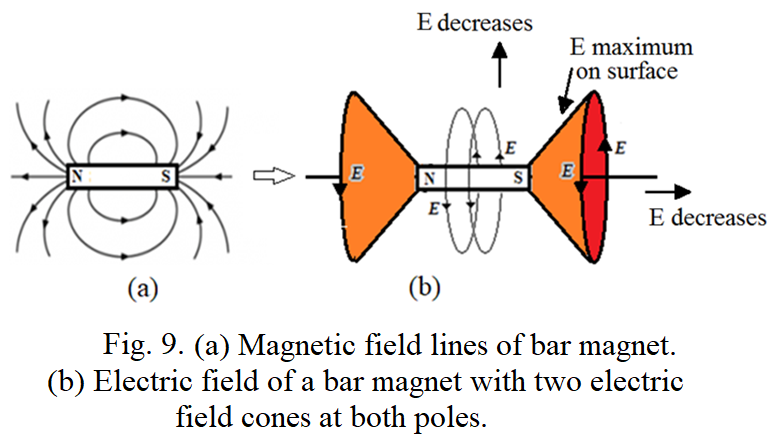In sequence of this discussion, as a straight long current produces parallel electric field, a bar magnet should produce circular electric field as illustrated in figure 9 having two electric field cones at both poles of the magnet. The electric field strength is maximum on the surface of the cone and it increases away from center. The electric field between the two poles of magnets in figure 8 is represented in figure 10 which increases away from the center. Deflection of electron beam using this circular electric field is explained below.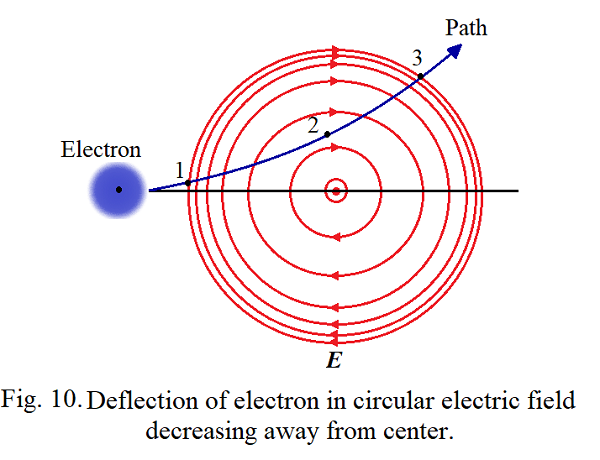Consider one electron in the electron beam of cathode ray tube (CRT) moving towards the screen with particular velocity through the electric field as represented in figure 10. This electric field applies asymmetric electric force on the electron which should be explained in terms of field-field interaction. The electric force on the electron’s field is in opposite direction of the circular electric field. At position 1, the electron’s electric field can be divided into two hemispheres, back and front. Force on the back field of the electron is more than the force on the front field because of which the electron turns in upward direction as it has forward velocity. At position 2, the electron’s field is to be again divide into lower and upper hemispheres. The upper field of the electron experiences greater force than its lower field because of which the electron turns in upward directionagain. At position 3, the electron’s field can be again divided into two hemispheres, front and back. The front field of the electron experiences more force than its back field because of which its moves again in upward direction. As all the electrons in the beam of CRT possess same velocity, they will travel along same path producing a sharp spot on the screen.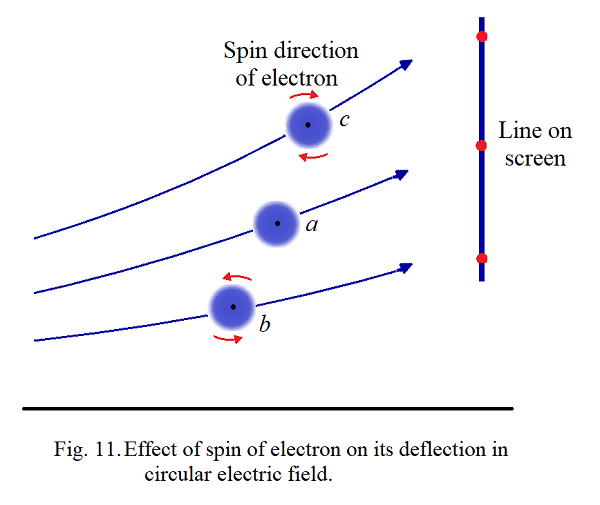In this explanation, the intrinsic spin of the electron is not taken into account and is represented by the curve ‘a’ in figure 11. If the electron possesses intrinsic spin then it can affect the deflection of the electron. Suppose it has clockwise spin as indicated in figure 11(b), then the strongest force on the electron’s field is in opposite direction of the spin motion because of which the electron will be deflected more in upward direction or towards the strong field region as indicated by curve ‘c’. If the electron spin is in anticlockwise direction then the strongest force on the electron’s field is in the direction of the spin because of which the electron will be deflected less as shown by curve ‘b’.The electrons in the electron beam may have randomly oriented spin because of which a line should be produced on the screen, as indicated in figure 4, which is not found experimentally indicating that the electrons in the electron beam do not possess any spin.Consequently, when electrons become free from their atoms they lose their spin implying spin is not intrinsic property of electrons. Therefore, the electrons may be acquiring spin through the need of arranging them in atoms. Thus, in distribution of the electrons in atoms, the electron’s spin should play important role.To understand it, the levitation of superconductor is to be understand properly as explained below.

#### Levitation of superconductor:

The levitation of superconductor in terms of asymmetric electric force applied by the circular electric field of bar magnet is illustrated in figure 12. The circular electric field of bar magnet, which decreases away from the magnet, produced electric current in the superconductor in the direction of the field. Therefore, the electric field of the magnet tries to push the electrons in the conductor, contributing the current, into the weak field region or away from the magnet. In similar way, the electric current in the superconductor produces circular electric field in opposite direction of the current which tries to increase the current in the magnet produced by unpaired electronsbecause of which the unpaired electrons in the magnet get pushed away from the superconductor. In this way a repulsion between magnet and superconductor is found which is considered as superconductor acts as a diamagnetic substance.If we place a superconductor on a magnet as illustrated in figure 12, then it will get levitated because of the repulsion between them. By increasing the distance between them, the repulsive force between them decreases as the strength of the electric field of the magnet linked to the superconductor decreases which causes to decrease the current produced in the superconductor. Similarly by decreasing the distance between the magnet and superconductor, the repulsive force between them increases as the strength of the electric field of the magnet linked to the superconductor increases which causes to increase the current produced in the superconductor.The gravitational force on the superconductor is constant therefore, the distance between the superconductor and magnet will get adjusted until the repulsive force between them and the gravitational force on the superconductor get balanced resulting into levitation of the superconductor. Thus the current produced in the superconductor is reached to the value such that the repulsive force and the gravitational force are balanced.Such things should also happen while rearranging the electrons in atoms.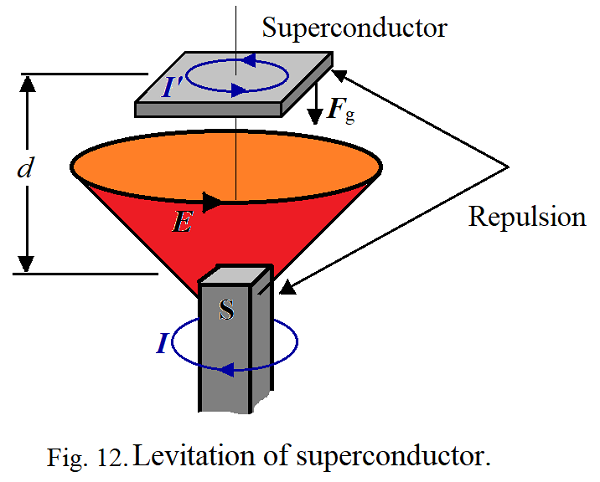#### Atomic model of helium:

Helium atom consists only two electrons, therefore it will be a simplest atomic mode to understand the role of spin of electrons.Generally electron has spherical symmetric electric field around itself. When such two electrons are brought close to each other, they will apply maximum force on each other to repel. Due to spin motion of an electron its electric field gets modified such that it has non-zero circular component about the axis of the spin. Increase in spin velocity should cause to increase in circular component of the electric field. Therefore, when two electrons which are in spin motion about same axis are brought close to each other, the circular electric field component of one electron will produce force on other electron in such a way that it will get spin motion in opposite direction of the first.This causes to reduce the net repulsion between them. Increase in spin velocity should cause further decrees in repulsion (Fig. 13.).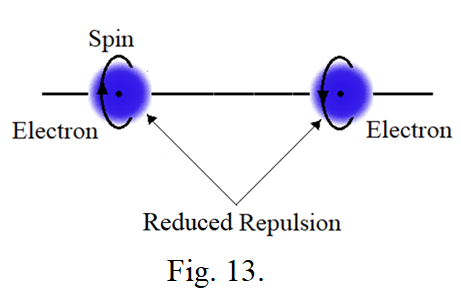In helium atom, there are two electrons. They can be arranged on opposite side of the nucleus such that the nucleus attracts them and the electrons themselves to repel each other. To reduce the repulsion between them, the electrons will be triggered to have spin motion in opposite direction of each other. Further the spin velocity of the electrons will be adjusted such that the repulsion between them and the attraction between the nucleus and the electrons will be balanced. If the electrons are more close to each other, high spin velocity will be required to reduce the repulsion. If the electron spin is quantized means it can have only certain values then the distance of the electron from nucleus in atoms will also be quantized. Thus, the electrons, in atoms, have no need to revolve in orbits to maintain their particular distance from nucleus as stated by Bohr. Further they are not behaving as waves as proposed by de Broglie. Using their spin motion, they can maintain a particular or safe distance from the nucleus in atoms. Thus, spin is not intrinsic property of electrons. A simple atomic model for helium atom is shown in figure 14.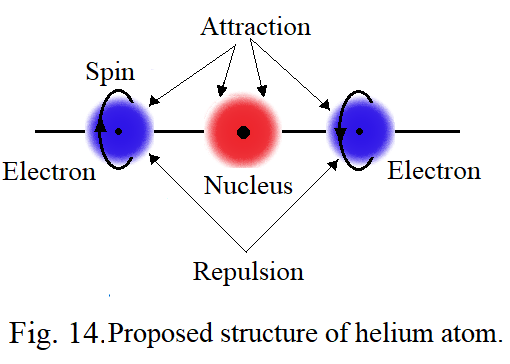#### On charged particles:

It is difficult to understand why and how the charged particles are created and why they are applying fore on each other. What is need of the space, because of which the charged particles are behaving like thus, is very important to understand. For that it is important to look in what wav we think about the field, especially about electric field, around a charged particle. The field is spherical symmetric around charged particles and can get propagated in terms of waves in space. It implies that the electric field should be simply a pressure. To create a pressure and propagation of that pressure requires an elastic medium. It means the space should be filled with that medium which was already considered as an aether but could not be proved. What we imagine about aether decides how to prove it. At present we keep the subject aside. Propagation of electric field in terms waves indicates that the field is simply a pressure. Electron has electric field and is spherical symmetric implies that the pressure should be either inward or outward. If we consider the pressure is outward or say negative electric field, then there should be other particles having inward pressure or positive electric field. These particles are protons. How a pressure about a point can be created in an elastic medium. Either something has to be removed or something has to be placed at that point. There is nothing to remove or nothing to place. Obviously a part of medium has to be removed which will make a pressure about the removed place or point in inward direction or creates positive electric field around that point which we are considering as a positive charged particle. The removed part of the medium has to be placed somewhere in the medium which creates outward pressure at that point or negative electric field about that point which we are considering as a negative charged particle. In nature we found that the electric field of electrons is quantized means having fixed value indicating a particular portion or fixed portion of the medium is removed every time when electrons are created. Creation of electrons causes to produce a hollow point at the place where a portion of the medium is removed. That point will have inward pressure or positive electric field which can be the proton. It implies that a neutral particle should not be existed in the universe. Then what are the neutrons is a big question. Further we know that a neutron can disintegrate into an electron and a proton indicating that it can be combination of an electron and a proton. The neutron should be a point in the medium where an electron and a proton are coupled to each other but not able to merge into each other to produce the point neutral once again. The tendency of the medium should be such that it should always try to keep the medium neutral. Two charges of same kind when come close to each other the field strength increases or the pressure increases because of which the medium tries to keep them away from each other. This we consider as repulsion between same kinds of charges. Two charges of opposite kinds when come close to each other the field strength decreases or the pressure reduces because of which the medium tries to bring them close to each other. This we consider as attraction between opposite kinds of charges. Oscillations of any kind of charges will produce disturbances in the medium which will propagate as a wave in the medium with fixed velocity as the medium is elastic. Further nothing is there like an electric charge. We experience only electric fields through the electric forces. Then there is another question, what is mass? A force required to move the charged particle will decide what the inertial mass of the particle is but actually mass like nothing is there. Inertial mas of electron is very less than inertial mas of proton and is to be understand properly. Further the charged particles possess gravitational mass too. Actually the gravitational mass and inertial mass of any particle is the same. The gravitational mass is experienced because of there is always a weak attraction between the particles whatever may be the kind of the field around them or kind of the chargeon them. If electric field is responsible to produce the forces between charged particles then why the gravitational force is also existed is beyond imagination. At this situation one may think that it may be arising because of imbalance between attractive and repulsive forces between the charged particles. Attractive force may be slightly greater than the repulsive force. This supports to the bending light ray near massive objects. On advent of this discussion, one important question remains to be discussed which is, can mass get converted into energy or can mass come to end? Actually mass like nothing should be there. Formation of neutron by coupling of a proton and an electron suggests that it should be difficult for mass to come to end otherwise neutrons should not be existed. While formation of neutron, the motions acquired by the proton and the electron should create disturbances in the medium which we may feel as creation of energy. Effective mass of the neutron is found to be less than the sum of individual masses of the electron and the proton. That is why we think that the mass is converted into energy. If we make a neutron to disintegrate into an electron and a proton by applying sufficient force, the individual particles will have usual masses. Thus the mass should not come to end. Survival of electrons and protonsonly in the universe indicates that charged particles different from protons and electronscannot not besurvived though we are able to create them.This part of discussion is simply a speculation.

#### Discussion:

Experimental confirmation of increase in magnetic force with increase in frequency of electromagnetic (EM) waves will indicates that the magnetic force can be responsible to produce the photoelectric effect. Thus EM waves need not to behave as particles consequently particles cannot be behaving as waves. Therefore, electrons in atoms may not be behaving as waves. On advent of this investigation, how the electrons in atoms can keep their safe distance from the nucleus becomes an essential part of investigation. Above investigation suggests that the spin of electrons plays important role in this regard. Opposite spin between two electrons reduces repulsion between them. Thus by acquiring spin motions, electrons can adjust their safe distance from the nucleus with balancing the attractive force between electrons and nucleus and the repulsive force between the electrons.However, this explanation has limitations and is applicable for helium atom only as it has only two electrons. If spin velocity of electrons can have particular or fixed values then the redial distance of the electron in atoms can have particular values too implying quantum situation. In atoms when unpaired electrons are present, then how the unpaired electrons acquire spin motion is a part of further investigation. In such cases the spin motion of other electrons in the atom can be responsible to produce spin motion of the unpaired electrons. It is very difficult to understand. However electrons are not revolving in atoms and not behaving as waves should be the fact. They can maintain their safe distance from the nucleus, otherwise, how matter can acquire a particular definite shape. If electrons are behaving as waves or revolving in atoms, then it will be impossible to acquire a particular and definite shape for matters (Fig. 15). It will be difficult to form molecules and compounds for atoms.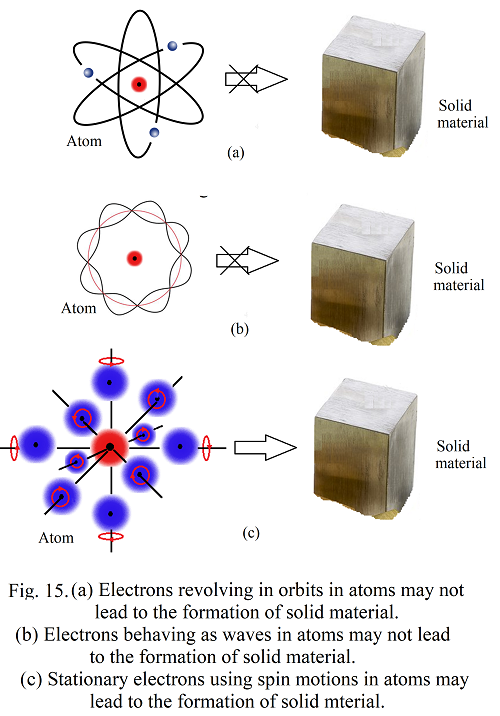Further, it is found that, a sodium atom emits a light wave consisting 650 wavelengths approximately. It implies that the corresponding electron in the atom should oscillate 650 times while producing the light wave with frequency of the wave. Further it should oscillate at particular and fixed position in the atom. Consequently the electron should not be revolved or acted as a wave in the atom.

#### Conclusion:

Electrons in atoms may not be circulating in stationary orbits about the nucleus as proposed by Bohr or may not be behaving as waves as proposed by Schrodinger. They can maintain their safe distance from the nucleus by adjusting their spin motions. Spin is not an intrinsic property of electrons. It is acquired by them through the need of distribution in atoms. Ability of electrons to keep safe distance from nucleus by adjusting their spin motions in atoms without revolving around the nucleus makes possible the atoms to form molecules, compounds and solid materials.

#### Reference:

1. G. H. Jadhav, ‘On Atomic Structure’, Submitted to IJSRPAS on 31st Oct 2021 with Paper ID-ISROSET-IJSRPAS-P-06836.

#### Location:

Dept. of Physics
Shri Chhatrapati Shivaji College, Omerga-413606, India

#### Call:

+91 9421359902